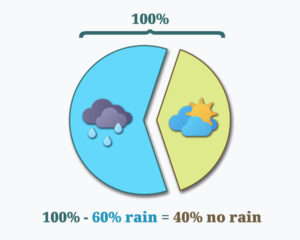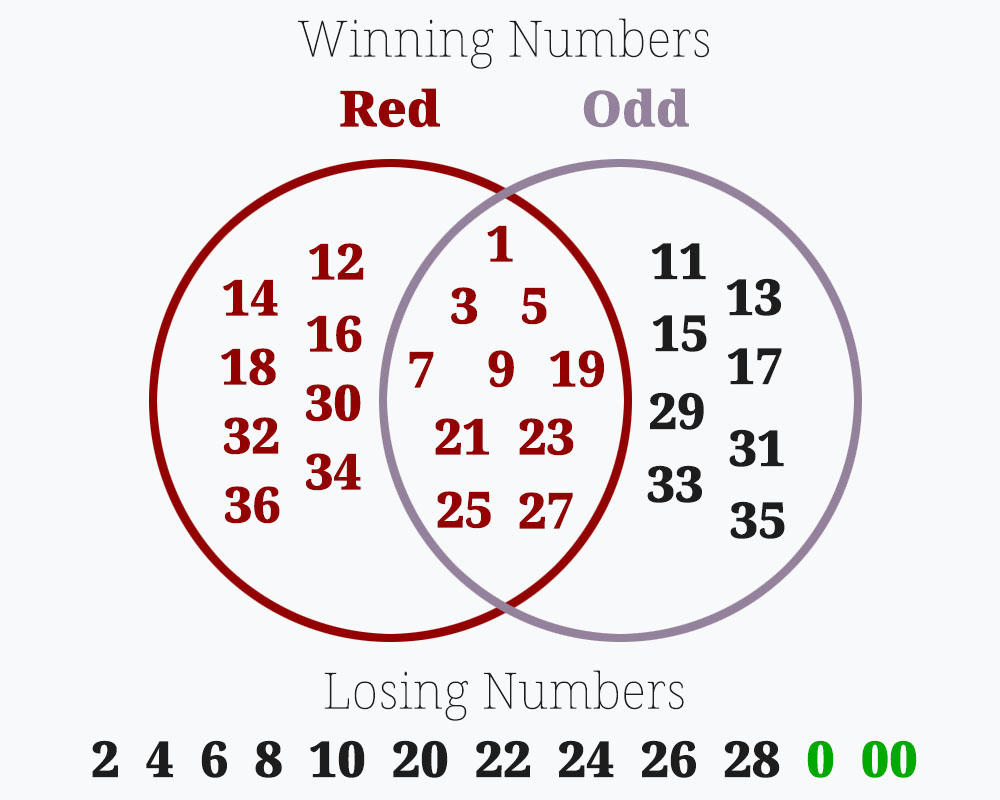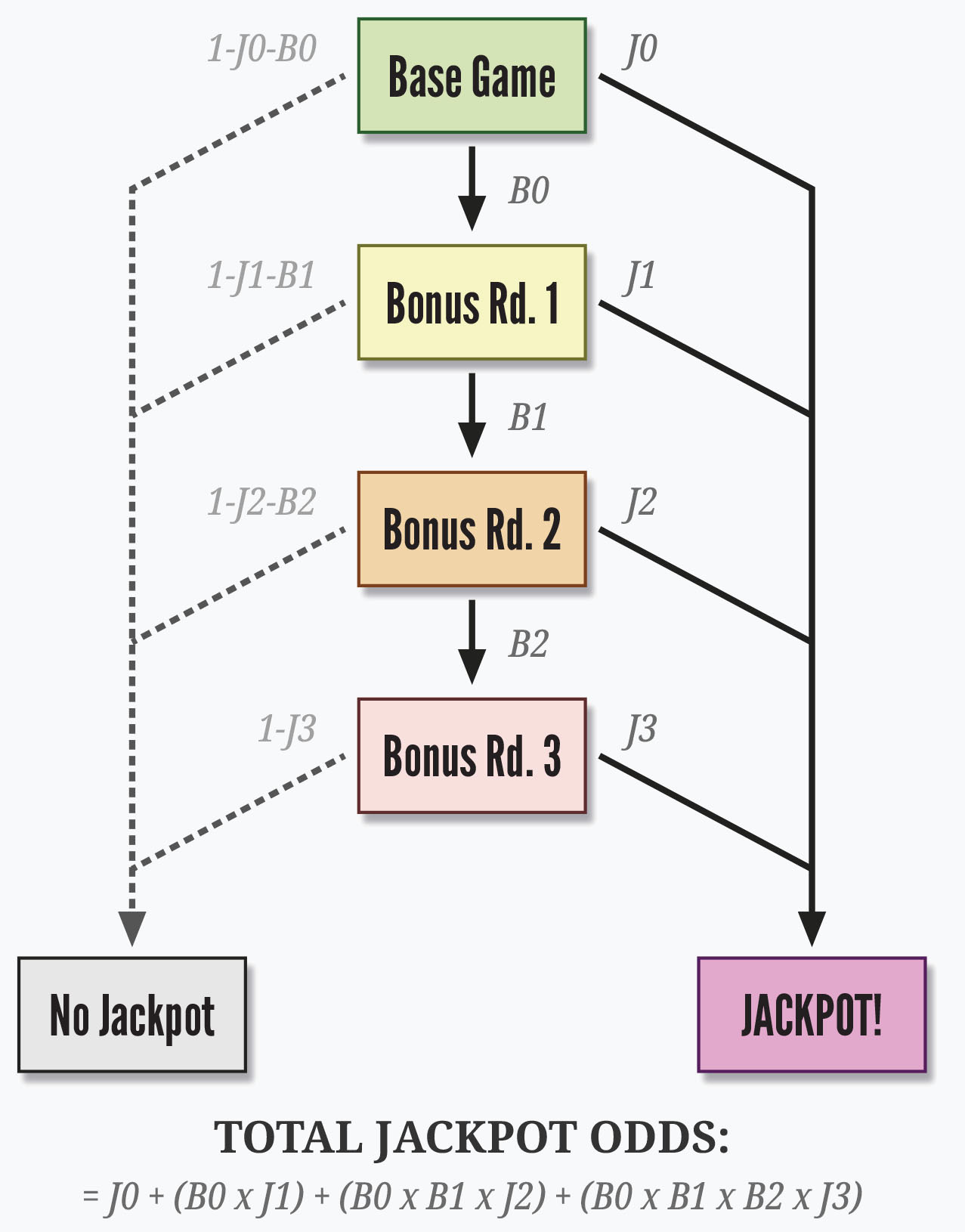# Calculating Probability for Casino Games

Bonus.com is an independent gambling news and information service. Bonus.com has partnerships with some of the top legal and licensed gambling companies in the US. When you claim a bonus offer or promotion through a link on this site, Bonus.com may receive referral compensation from the gambling company. Although the relationships we have with gambling companies may influence the order in which we place companies on the site, all reviews, recommendations, and opinions are wholly our own. They are the recommendations from our authors and contributors who are avid casino players and sports fans themselves.

Bonus.com is licensed and regulated to operate in AZ, CO, CT, IL, IN, KS, LA, MI, NJ, NY, PA, TN, and VA.

Sometimes, you may not know the probability of the event you’re wondering about. However, if you know some other related probabilities, you might be able to calculate it.

Probability is a branch of mathematics in its own right. It’s simpler than some of the others, but still not a topic we can explore exhaustively in a few short articles.

That said, there are some basic tools we can teach you for using simple probabilities to calculate more complicated ones. In fact, there’s a lot you can do just by combining three basic rules in various ways:

• All possible outcomes for a single attempt must add up to 100% probability.
• To get the chance of two independent events both happening, multiply their probabilities.
• When there are multiple ways to get the same result on the same attempt, add the probabilities.

These will allow you to answer questions like:

• If I make several roulette bets at once, what are the chances that I miss all of them?
• What are the odds of getting dealt a blackjack once? What about three times in a row?
• How likely am I to crap out within the first four rolls?

## Probabilities Always Add Up to 100%Going back to our first lesson, a percentage probability tells you how many times, on average, something will happen out of 100 tries.

There’s no such thing as “no result” in probability. Even nothing happening is a type of result. So, if we try something 100 times, we get 100 results.

That means if we add up all the possible things that can happen, the percentages have to add up to 100%.

This is easiest to see when there are only two options. In these cases, you can easily reverse a probability by subtracting it from 100%. That gives you the probability of the opposite outcome.

For instance, a 60% chance of rain means a 40% chance of no rain.

The same is true when the probability is “out of” something other than 100. For instance, there are 38 numbers on an American roulette wheel. If you count all the winning numbers for your bets, you can subtract that from 38 to give you the number of losers.

### Don’t Count Anything Twice!However, it’s important in these cases not to count anything twice.

Using the roulette example, let’s say we bet on Red and on Odd. There are 18 Red numbers and 18 Odd numbers on the wheel. However, 10 of those numbers are both Red and Odd. (Not nine, as you might expect. Each color on a roulette wheel isn’t split evenly between odd and even!)

If we’re adding the Red and Odd numbers together, we have to subtract the ones we’ve double-counted. So, the number of winning spaces is 18 + 18 – 10 = 26.

Another way of looking at it is that we have:

• Eight Red numbers that aren’t Odd
• Eight Odd numbers that aren’t Red
• 10 numbers that are both Red and Odd

You can see that adding those up gives us the same result: 9 + 9 + 9 = 27.

Either way, the number of losing spaces is 38 minus 27, or 11. Therefore, our probability of losing both bets is 11 in 38, or 29%.

When dealing with the probabilities of several events at once, the first thing you need to ask yourself is whether they’re dependent or independent events. That is, does the probability of the second one change after you know the outcome of the first?

Fortunately, probabilities for casino games are usually independent, which makes the math much easier.

For instance, one roll of the dice or one spin of a slot machine does not affect the next one in any way. It doesn’t matter if the roulette wheel has just landed on Black five times in a row: the next spin is equally likely to be Red or Black. (To believe otherwise is the Gambler’s Fallacy, which we discussed in the first lesson.)

The real world is usually more complicated. For instance, investors react to the ups and downs of the stock market and also to their beliefs about what everyone else is thinking. So, what happens to share prices on one day can affect what happens the next. (But if you could figure out exactly how that works, you would become very rich.)

At the end of the article, we’ll take a little look at dependent probabilities. But our basic tools assume that the probabilities are independent.

## Probability of Succeeding on All Attempts

Sometimes, we know the probability of something happening once but want to know the probability that it happens several times in a row. Or we might know the probabilities of several different things and want to know the chances that they all happen.

The rule for this situation is that we multiply the chances together. Say that your casino is offering 1 out of every 20 guests (5%) a spin on a prize wheel as a promotion. That wheel has ten equal spaces (10% chance of each), and the top prize is \$500 in cash.

Your chances of winning \$500 are the probability of being chosen (5%) times the probability of a lucky spin (10%). So, that’s 5% x 10% = 0.5%, or a one in 200 chance.

A special case of this is when you want to know the odds of the same thing happening several times in a row. In that case, the probability is the same each time. So, you can just square the probability for the chance of the thing happening twice in a row, cube it for three times in a row, etc.

For instance, the probability of getting dealt a natural blackjack from a single deck is around 4.8%. (We’ll calculate that ourselves in the final section!)

Knowing that, you can find the odds of getting dealt several blackjacks in a row.

• One blackjack: 0.0481 = 0.048 (4.8%, or about 1 in 21)
• Two blackjacks in a row: 0.0482 = 0.0023 (0.23%, or about 1 in 434)
• Three blackjacks in a row: 0.0483 = 0.00011 (0.011%, or about 1 in 9042)
• etc.

Remember: This only works when the probabilities are independent. Maybe you know the chance of rain tomorrow and the probability of seeing your friend at the park. But you can’t just multiply them together to get the odds of meeting your friend in the rain since your friend is more likely to stay home on a rainy day than a sunny one!

## Probability of Succeeding on at Least One Attempt

You can solve many other probability problems by applying these two rules in different combinations.

For instance, let’s say we try something several times and want to know the chance of getting a result at least once.

The key is to remember that we can reverse probabilities by subtracting them from 100%.

1. First, we reverse the chance of hitting our result to get the chance of missing it.
2. Then we calculate the probability of missing it several times in a row.
3. Finally, we reverse that again because hitting at least once is the same as not missing every time.

For instance, when playing craps, your chance of crapping out (rolling a 7) is 1 in 6 for each roll. That means the chance of not crapping out is 5 in 6. (For simplicity, we’re ignoring the come out roll which has different odds.)

Just like last time, we’re going to multiply those probabilities together to get the chance of failing several times in a row.

• One roll without crapping out: 0.8331 = 0.833 (83.3%)
• Two rolls without crapping out: 0.8332 = 0.694 (69.4%)
• Three rolls without crapping out: 0.8333 = 0.578 (57.8%)
• Four rolls without crapping out: 0.8334 = 0.481 (48.1%)

But if we want to know how likely it is that we have crapped out within a certain number of rolls, we have to reverse that probability.

• Crapping out on the first roll: 0.8331 = 0.833 (83.3%)
• Crapping out within two rolls: 0.8332 = 0.694 (69.4%)
• Crapping out within three rolls: 0.8333 = 0.578 (57.8%)
• Crapping out within four rolls: 0.8334 = 0.481 (48.1%)

## Solving Complex Scenarios With Probability Trees

Sometimes, there is more than one way to get to a result. In these cases, we can calculate each path separately and add them together.

For instance, to get a natural blackjack, we need an Ace plus any card with a value of ten. But this could happen in either order.

The odds of getting an Ace on the first card are 4 in 52 (7.7%).

After that, there are 51 cards left if we’re playing with a single deck. Sixteen of those have a value of ten (four Tens, four Jacks, four Queens and four Kings). That’s 16 in 51 (31.4%).

Multiplying those probabilities gives us a 2.4% chance of getting the blackjack with the Ace first.

4/52 x 16/51 = 2.4%

What about the other way around? We start with a 16 in 52 chance of getting ten, then a 4 in 51 chance of getting an Ace. Astute readers might notice that this is going to come out to 2.4% again because of symmetry.

16/52 x 4/51 = 2.4%

Adding those together gets us 4.8% as the combined chance of getting a blackjack either way. Coincidentally, that turns out to be roughly 1 in 21, the very number we’re shooting for!

2.4% + 2.4% = 4.8% = about 1 in 21

This method is called a probability tree because of how it looks when there are many branches. There’s no limit to how many paths you can look at or how many steps they involve. They won’t always be symmetrical like our blackjack example.

To keep things simple, you can merge the branches that lead to the same outcome. Just multiply all the probabilities along every path that can get you to the outcome you’re looking for, and add them up.

For instance, imagine a slot machine with a three-stage bonus game offering ever-increasing chances to win the jackpot. That might look like this:## Casino School: Probability

This has been Probability Lesson 4, which concludes our four-part Casino School series. Catch up on what you missed: